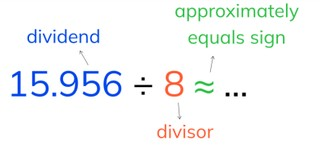Estimated division with decimal numbers with 3 or more decimal places

# Estimated division with decimal numbers with 3 or more decimal places

Students learn estimated division with decimal numbers.8,000 schools use Gynzy92,000 teachers use Gynzy1,600,000 students use Gynzy

## General

The students learn estimated division with decimal numbers with 3 or more decimal places.

## Standards

CCSS.Math.Content.6.NS.B.3

## Learning objective

Students will be able to estimate quotients with decimal numbers with 3 or more decimal places.

## Introduction

You have the students practice rounding, by asking them which pond the swan should be dragged to. Also ask how they know this.

## Instruction

First you explain what the dividend, the divisor, and the approximately equals sign are. Then you tell the students that with estimated division you always look for a dividend that is divisible by the divisor. Next you show estimation step by step. Explain that you first think of which numbers are divisible by the divisor. Find the outcome that is the closest to the dividend. After that you can solve the problem. Explain that you need to stay as close as possible to the dividend. This way, you will be closer to the exact answer. After this the students practice choosing the correct estimated quotient. Also explain why certain estimated division problems are not possible, because they will not give a whole number (for example, you can't round 68.911 ÷ 6 to 70 ÷ 6, but you can round it is 66 ÷ 6). Next the students are going to estimate quotients on their own. For this, explain that sometimes you also need to round the divisor, if it is also a decimal number. For this, start by looking for a good estimated division problem. Show this step by step. After that, have the students estimate division problems on their own, and ask how they rounded. Next discuss whether the exact answers are more or less than the estimate. Have the students reason how you can know this. Then recall the meaning of the symbols < and >.

Check whether the students can estimate quotients by asking them the following question:
- What do you do with the decimal number if you want to estimate a quotient?

## Quiz

The students test their understanding of estimated division with decimal numbers with 3 or more decimal places, through ten exercises. In some of the exercises, they much choose the correct estimated division problem. In others, they must make an estimate of the answer.

## Closing

You discuss once again with the students that it is important to be able to estimate quotients with decimal numbers, because then you quickly know approximately how much you can divide a number by. Check whether the students can estimate quotients by dragging the problems to the correct boxes.

## Teaching tips

When students have difficulty with estimated division with decimal numbers with 3 or more decimal places, first practice finding a good estimated division problem. To do this, have the students write down the outcomes of the table of the divisor.

### The online teaching platform for interactive whiteboards and displays in schools

• Save time building lessons

• Manage the classroom more efficiently

• Increase student engagement